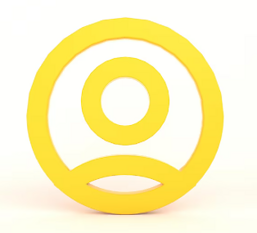(5/5)

### Consider a 2-D grid with 5x5=25 rooms, as shown the agent knows the environment and dirt distribution this is a fully observable problem.

INSTRUCTIONS TO CANDIDATES

Consider a 2-D 25-room vacuum-cleaner world as follows:

• The world is a 2-D grid with 5x5=25 rooms, as shown The agent knows the environment and dirt distribution. This is a fully observable problem.
• The agent can choose to move left (Left), move right (Right), move up (UP), move down (DOWN), suck up the dirt (Suck), or do nothing (NoOp). Clean rooms stay clean. The agent can’t go outside the environment, i.e. the actions to bring the agent outside the environment are not
• Performance measure:
1. 4 point for each cleaned up room (changing the room from dirty to clean)
2. -1 point for Left
3. -1.1 point for Right
4. -1.2 point for UP
5. -1.3 point for DOWN
6. -0.2 point for Suck
7. 0 point for NoOp

Over a lifetime of 10 time steps. Higher performance points are better.

 (1,1) (1,2) (1,3) (1,4) (1,5) (2,1) (2,2) … (3,1) … (4,1) (5,1) (5,5)

In this programming assignment, you should implement the following 4 algorithms to solve the 2-D 25-room vacuum-cleaner world problem:

1. uniform cost tree search, up to search tree depth 10,
2. uniform cost graph search, up to search tree depth 10,
3. depth-limited depth-first tree search, with depth limit 10,
4. depth-limited depth-first graph search,with depth limit

Follow the Tree-Search and Graph-Search pseudocode in the lecture slides (copied below), but removing the Goal-Test. You need to search the whole tree and return the best solution found. Breaking ties of search nodes randomly.

function Tree-Search(problem, fringe) returns a solution, or failure fringe = Insert(Make-Node(Initial-State[problem]), fringe) loop do

if fringe is empty then return failure

node = Remove-Front(fringe)

if Goal-Test(problem,State(node)) then return node fringe = InsertAll(Expand(node, problem), fringe)

end

function Graph-Search(problem, fringe) returns a solution, or failure closed = an empty set

fringe = Insert(Make-Node(Initial-State[problem]), fringe) loop do

if fringe is empty then return failure node = Remove-Front(fringe)

if Goal-Test(problem,State[node]) then return node if State[node] is not in closed then

fringe = InsertAll(Expand(node, problem), fringe)

end

(5/5)

## Related Questions

##### CSI 1420 Introduction to C Programming & Unix Fall 2018, CRN 44882, Oakland University Homework Assignment 6 - Using Arrays and Functions in C

DescriptionIn this final assignment, the students will demonstrate their ability to apply two majorconstructs of the C programming language – Fu

##### The standard path finding involves finding the (shortest) path from an origin to a destination, typically on a map. This is an

Path finding involves finding a path from A to B. Typically we want the path to have certain properties,such as being the shortest or to avoid going t

##### Develop a program to emulate a purchase transaction at a retail store. This program will have two classes, a LineItem class and a Transaction class. The LineItem class will represent an individual

Develop a program to emulate a purchase transaction at a retail store. Thisprogram will have two classes, a LineItem class and a Transaction class. Th

##### SeaPort Project series For this set of projects for the course, we wish to simulate some of the aspects of a number of Sea Ports. Here are the classes and their instance variables we wish to define:

1 Project 1 Introduction - the SeaPort Project series For this set of projects for the course, we wish to simulate some of the aspects of a number of

##### Project 2 Introduction - the SeaPort Project series For this set of projects for the course, we wish to simulate some of the aspects of a number of Sea Ports. Here are the classes and their instance variables we wish to define:

1 Project 2 Introduction - the SeaPort Project series For this set of projects for the course, we wish to simulate some of the aspects of a number of

### Ask This Assignment To Be Done By Our ExpertsGet A+ Grade Solution Guaranteed##### joyComputer science
(4/5)